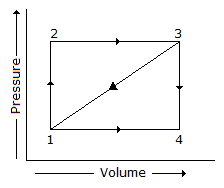1. A cycle consisting of two isothermal and two isentropic processes, is known as
2. In S. I. units, the value of the universal gas constant is
3. A path 1-2-3 is given. A system absorbs 100 kJ as heat and does 60 kJ of work while along the path 1-4-3, it does 20 kJ of work. The heat absorbed during the cycle 1-4-3 is4. When wood is heated with a limited supply of air to a temperature not less than 280°C, the resulting fuel is
5. The fuel mostly used in blast furnace for extracting pig iron from iron ores is
6. It is __________ to make an engine working on a Carnot cycle.
7. The __________ states that change of internal energy of a perfect gas is directly proportional to the change of temperature.
8. Which of the following is the extensive property of a thermodynamic system?
9. Steam coal is a
10. The atomic mass of sulphur is
11. Which of the following statement is correct?
12. When a perfect gas is expanded through an aperture of minute dimensions, the process is known as
13. In an ideal gas turbine plant, it is assumed that the compression and expansion processes are
14. Petrol is distilled at
15. First law of thermodynamics deals with
16. Second law of thermodynamics defines
17. The work done in a free expansion process is zero.
18. The ratio of root mean square velocity to average velocity of gas molecules at a particular temperature is
19. The efficiency of the Carnot cycle is (where T1 and T2 = Highest and lowest temperature during the cycle)
20. When gas is heated at constant pressure, the heat supplied is utilised in
Thermodynamics MCQ 12
You got {{userScore}} out of {{maxScore}} correct
{{title}}
{{image}}
{{content}}